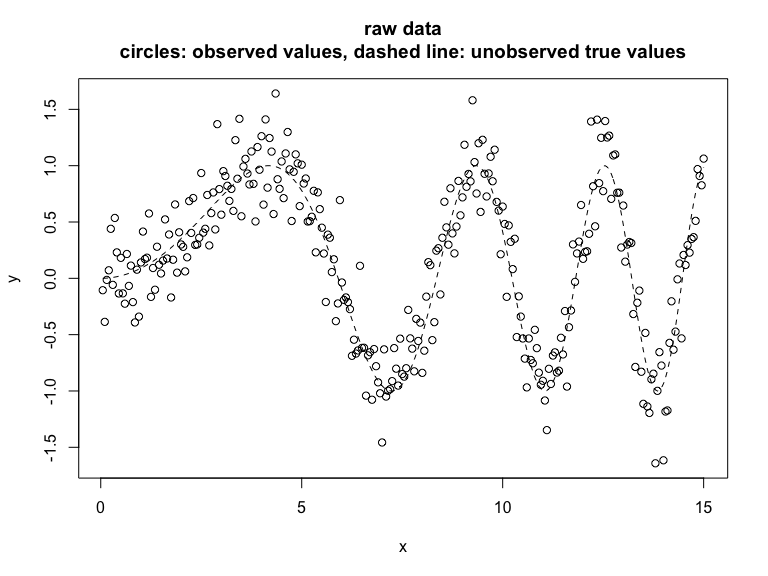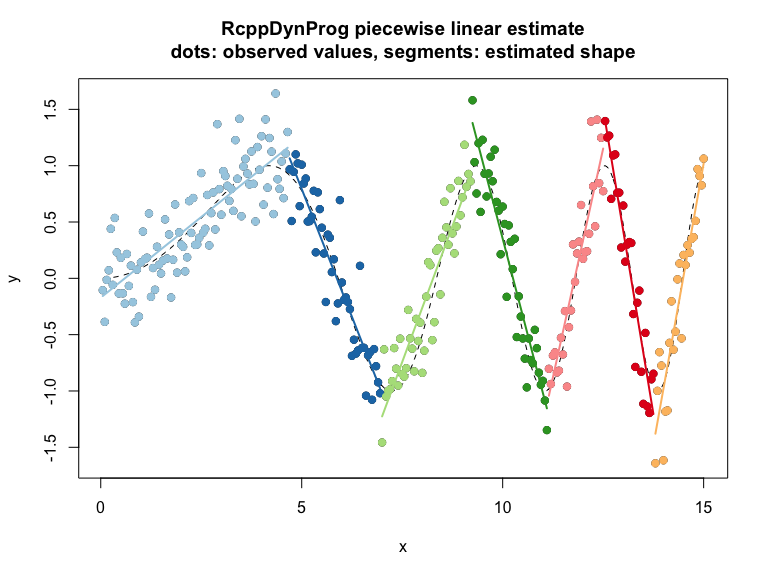# Linear Segmentation

#### 2023-08-19

In this example we fit a piecewise linear function to example data.
Please see here for a discussion of the methodology.

library("RcppDynProg")

set.seed(2018)
g <- 100
d <- data.frame(
x = 0.05*(1:(3*g))) # ordered in x
d$y_ideal <- sin((0.3*d$x)^2)
d$y_observed <- d$y_ideal + 0.25*rnorm(length(d$y_ideal)) # plot plot(d$x, d$y_observed, xlab = "x", ylab = "y", main = "raw data\ncircles: observed values, dashed line: unobserved true values") lines(d$x, d$y_ideal, type = "l", lty = "dashed")x_cuts <- solve_for_partition(d$x, d$y_observed, penalty = 1) print(x_cuts) ## x pred group what ## 1 0.05 -0.1570880 1 left ## 2 4.65 1.1593754 1 right ## 3 4.70 1.0653666 2 left ## 4 6.95 -0.9770792 2 right ## 5 7.00 -1.2254925 3 left ## 6 9.20 0.8971391 3 right ## 7 9.25 1.3792437 4 left ## 8 11.10 -1.1542021 4 right ## 9 11.15 -1.0418353 5 left ## 10 12.50 1.1519490 5 right ## 11 12.55 1.3964906 6 left ## 12 13.75 -1.2045219 6 right ## 13 13.80 -1.3791405 7 left ## 14 15.00 1.0195679 7 right d$estimate <- approx(x_cuts$x, x_cuts$pred, xout = d$x, method = "linear", rule = 2)$y
d$group <- as.character(findInterval(d$x, x_cuts[x_cuts$what=="left", "x"])) print(sum((d$y_observed - d$y_ideal)^2)) ##  20.42462 print(sum((d$estimate - d$y_ideal)^2)) ##  3.536541 print(sum((d$estimate - d$y_observed)^2)) ##  20.53796 # plot plot(d$x, d$y_observed, xlab = "x", ylab = "y", main = "RcppDynProg piecewise linear estimate\ndots: observed values, segments: estimated shape") points(d$x, d$y_ideal, type = "l", lty = "dashed") cmap <- c("#a6cee3", "#1f78b4", "#b2df8a", "#33a02c", "#fb9a99", "#e31a1c", "#fdbf6f", "#ff7f00", "#cab2d6", "#6a3d9a", "#ffff99", "#b15928") names(cmap) <- as.character(seq_len(length(cmap))) points(d$x, d$y_observed, col = cmap[d$group], pch=19)
groups <- sort(unique(d$group)) for(gi in groups) { di <- d[d$group==gi, , drop = FALSE]
lines(di$x, di$estimate, col = cmap[di\$group[]], lwd=2)
}